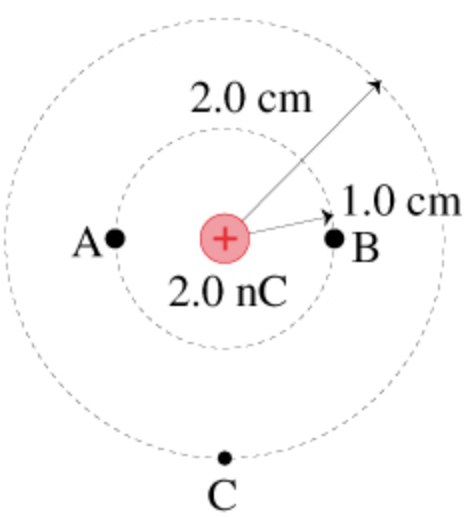In: Physics

# What is the potential difference ΔVAB?

What is the potential difference ΔVAB?## Solutions

##### Expert Solution

The points $$\mathrm{A}$$ and $$\mathrm{B}$$ are at the same distance from charge $$\mathrm{q} .$$
Hence, potential at $$\mathrm{A}$$ and $$\mathrm{B}$$ are same.

The potential due to a point charge at a distance $$r$$ is given by $$V=\frac{1}{4 \pi \varepsilon_{0}} \frac{q}{r}$$ Here, $$\frac{1}{4 \pi \varepsilon_{0}}=9 \times 10^{9} \mathrm{Nm}^{2} / \mathrm{C}^{2}$$
\begin{aligned} q=& 2.0 \mathrm{nC}=2.0 \times 10^{-9} \mathrm{C} \\ r=& 1.0 \mathrm{~cm}=1.0 \times 10^{-2} \mathrm{~m} \\ \text { Hence, } V_{A}=& V_{B}=\left(9 \times 10^{9} \mathrm{Nm}^{2} / \mathrm{C}^{2}\right) \frac{\left(2.0 \times 10^{-9} \mathrm{C}\right)}{\left(1.0 \times 10^{-2} \mathrm{~m}\right)} \\ &=1800 \mathrm{~V} \end{aligned}
Adjusted to two significant figures, $$V_{A}=V_{B}=1800 \mathrm{~V}$$ For $$\mathrm{C} r=2.0 \mathrm{~cm}=2.0 \times 10^{-2} \mathrm{~m}$$ Hence,
\begin{aligned} V_{C} &=\left(9 \times 10^{9} \mathrm{Nm}^{2} / \mathrm{C}^{2}\right) \frac{\left(2.0 \times 10^{-9} \mathrm{C}\right)}{\left(2.0 \times 10^{-2} \mathrm{~m}\right)} \\ &=900 \mathrm{~V} \end{aligned}

Adjusted to two significant figures, $$V_{C}=900 \mathrm{~V}$$

a) As $$\mathrm{A}$$ and $$\mathrm{B}$$ are at same potential, Hence, potential difference between $$\mathrm{A}$$ and $$\mathrm{B}$$ is $$\Delta V_{A B}=0$$

b) Potential Difference between the points $$\mathrm{B}$$ and $$\mathrm{C}$$ is
\begin{aligned} \Delta V_{B C} &=V_{B}-V_{C} \\ &=1800-900 \\ &=900 \mathrm{~V} \end{aligned}
Adjusted to two significant figures, $$\Delta V_{B C}=900 \mathrm{~V}$$